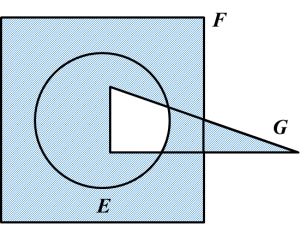# 4.5.5 Operations on Sets, SPM Paper 2 (Long Questions)

Question 9:
The Venn diagram in the answer space shows sets E, and G such that the universal set, $\xi =E\cup F\cup G.$
$\begin{array}{l}\left(a\right)\text{}E\text{}‘\cap G‘\\ \left(b\right)\text{}\left(E\cap F\right)‘\text{}\cup G‘\end{array}$